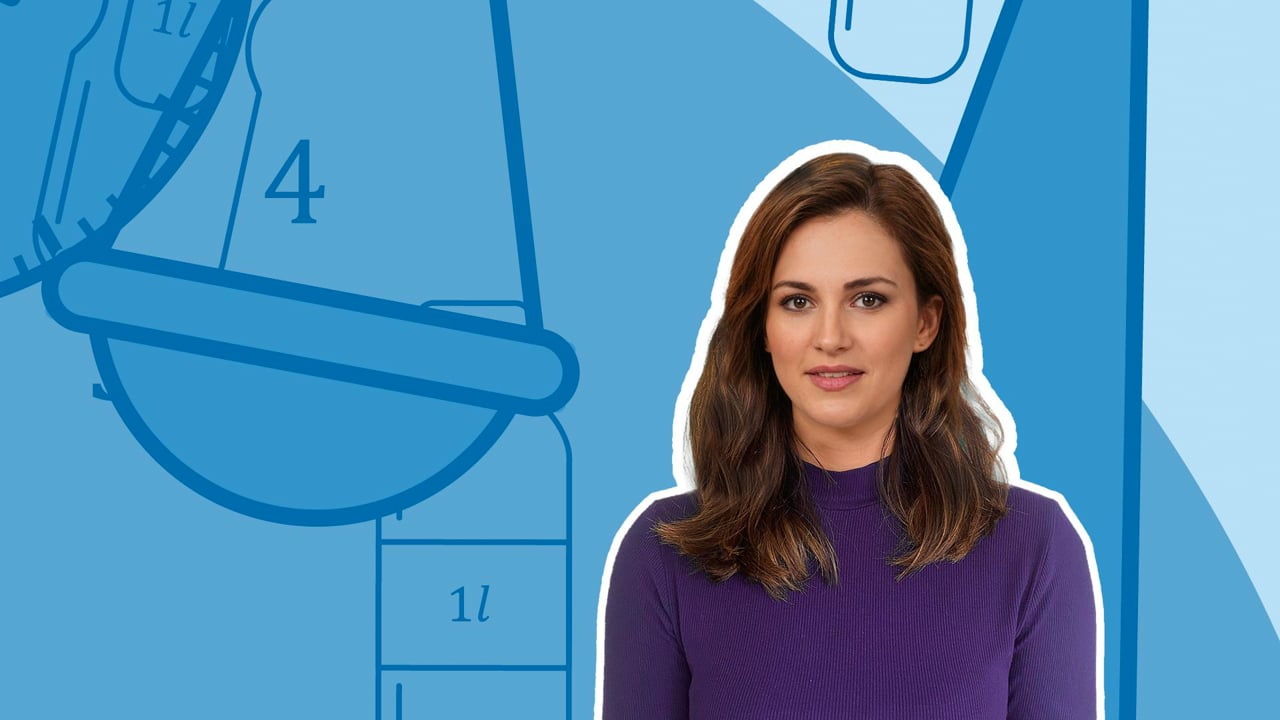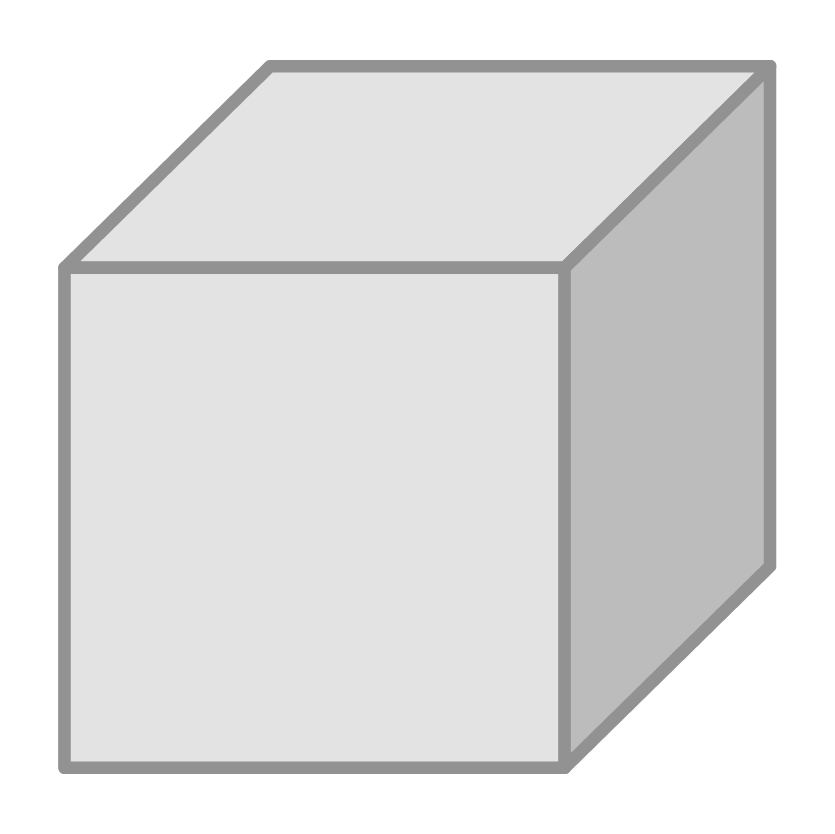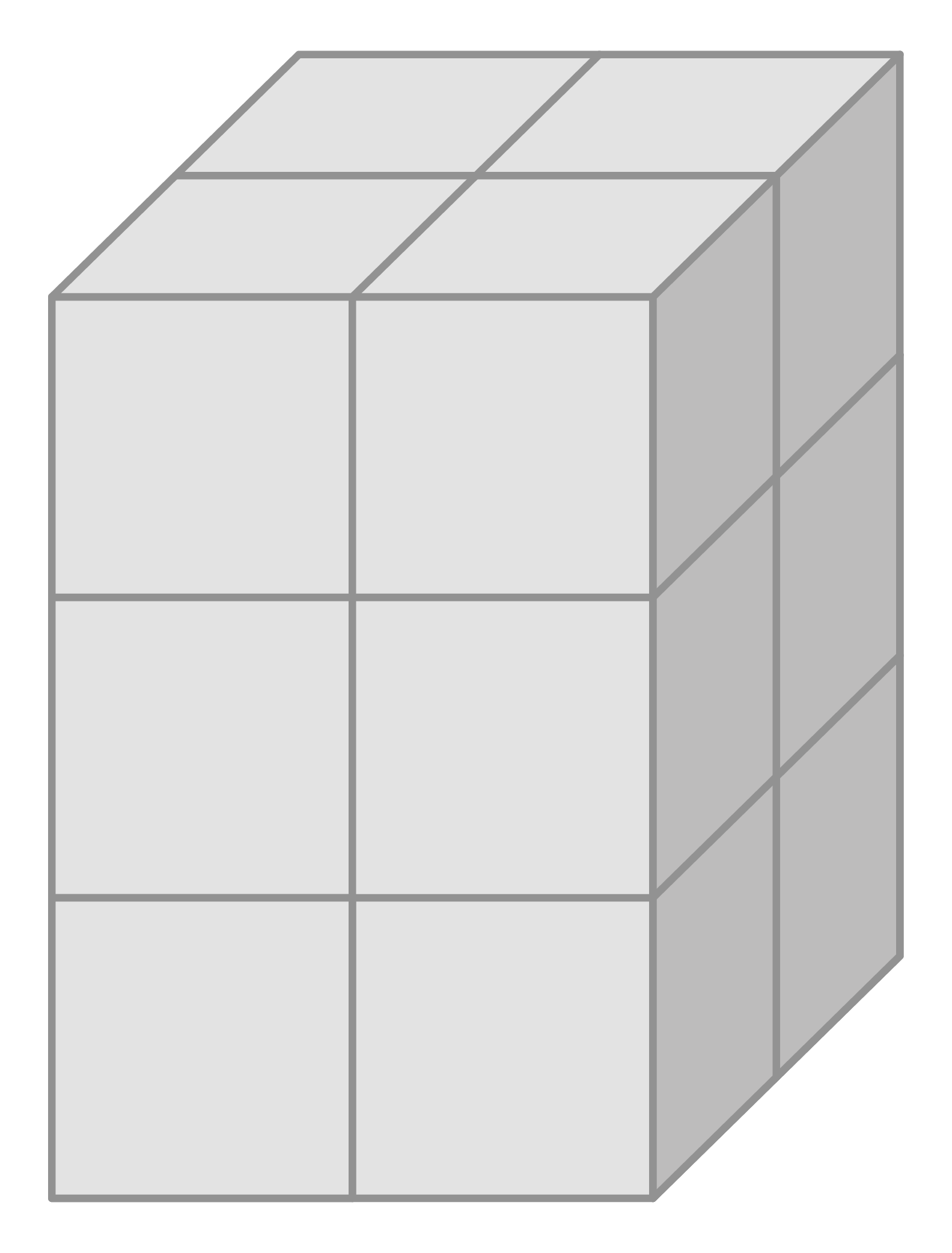Chapter OverviewMaths

Number and place value

Multiplication and division

Fractions

Measurement

Geometry - properties of shapes

Geometry - position and direction

Statistics

Maths

# Estimating volume and capacity0%

Summary

# Estimating volume and capacity

## ​​In a nutshell

The volume of a shape tells you how much space it takes up. You can measure the volume by counting squares or using a formula. Liquids also take up volume. Objects such as containers and jugs have a capacity.

## Volume

Volume is the measurement of how much space a 3D shape takes up. It can be measured in different units such $mm^3, cm^3$ and $m^3$​.

## Using cubes to find the volume

Cubes with a given length can be used to work out volume.

##### Example 1

Find the volume of the cuboid, by counting the cubes.

 ​$1 \ cube =1cm^3$​​Firstly, count the number of cubes in the cuboid.

The cuboid is made up of $12$​ cubes.

Since each cube is $1cm^3$ multiply this by $12$ to find the total volume.

The volume of the cuboid is $\underline{12cm^3}$.

## Using the formula to find the volume

The volume of a cuboid can be found using the following formula:

$V=l \times w \times h$​​
 ​$V$​​ The Volume of the cuboid. ​$l$​​ The length of the cuboid. ​$w$​​ The width of the cuboid. ​$h$​​ The height of the cuboid.
##### Example 2

Find the volume of the cuboid using the formula.

$height = 3cm, \ width = 2cm, \ length = 5cm$​​

Substitute the values for height, width and length in to the formula.

\begin{aligned}Volume &= l \times w \times h \\Volume &= 5 \times 2 \times 3 \\Volume &= \underline{30cm^3}\end{aligned}

## Capacity

Liquids also have a volume which takes up space in a given vessel, such as a jug. The jug's capacity is the maximum volume of liquid it may hold.

##### Example 3

Determine the capacity of the jug.

The jug is filled up to $1300ml$​. However, the maximum capacity of the jug is $1500ml$​.

The capacity of the jug is $\underline{1500ml}$.

FAQs

• Question: What is capacity?

Answer: Capacity is the maximum volume of liquid a vessel may hold.

• Question: What units is volume measured in?

Answer: Volume can be measured in different units such millimetres cubed, centimetres cubed and metres cubed.

• Question: What is volume?

Answer: Volume is the measurement of how much space a 3D shape takes up.

Theory

Exercises